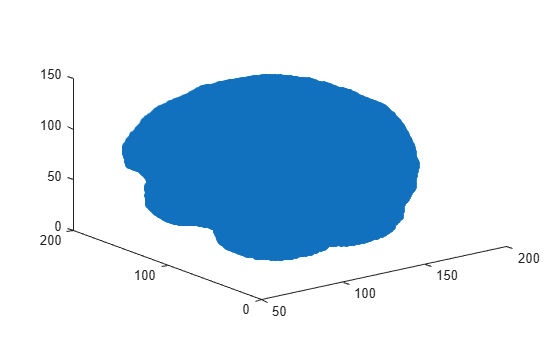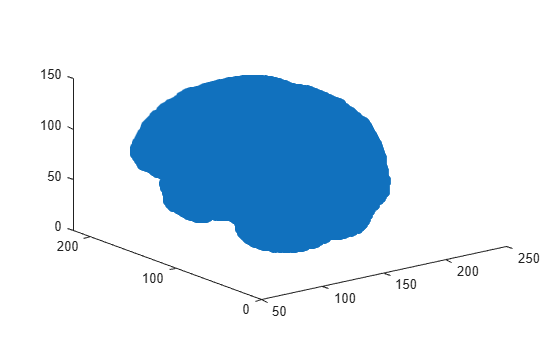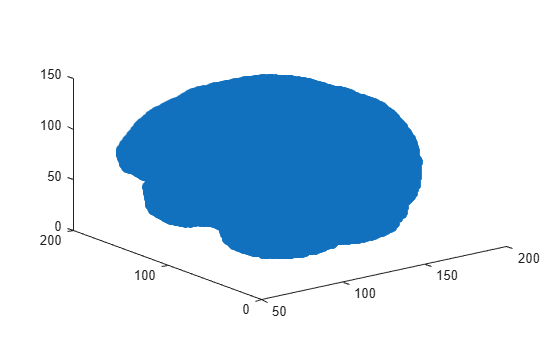# imregicp

Surface registration using iterative closest point algorithm

## Syntax

``regSurface = imregicp(movingSurface,fixedSurface)``
``regSurface = imregicp(movingSurface,fixedSurface,Name=Value)``
``[regSurface,tform] = imregicp(___)``
``[regSurface,tform,rmse] = imregicp(___)``

## Description

The `imregicp` function uses the iterative closest point (ICP) algorithm for rigid registration of surfaces. Use this function to register surfaces extracted from medical volumes.

````regSurface = imregicp(movingSurface,fixedSurface)` transforms the surface `movingSurface`, so that it is registered with the reference surface `fixedSurface` using the ICP algorithm. The function returns the registered surface `regSurface`.```

example

````regSurface = imregicp(movingSurface,fixedSurface,Name=Value)` specifies options for the ICP algorithm using one or more optional name-value arguments.```
````[regSurface,tform] = imregicp(___)` returns the transformation `tform` between the moving surface and the registered surface, in addition to any combination of input arguments from previous syntaxes.```
````[regSurface,tform,rmse] = imregicp(___)` returns the root mean squared error (RMSE) `rmse` of the Euclidean distance between the inlier points of the aligned surfaces `regSurface` and `fixedSurface`, in addition to any combination of input arguments from previous syntaxes.```

## Examples

collapse all

Load a MAT file containing intensity volume data into the workspace. Extract the volume data.

```load(fullfile(toolboxdir("images"),"imdata","BrainMRILabeled","images","vol_001.mat")); V = vol;```

Specify an isovalue for isosurface extraction.

`isovalue = 0.5;`

Extract the isosurface of the reference volume at the specified isovalue.

`[~,fixedSurface] = extractIsosurface(V,isovalue);`

Display and inspect the reference isosurface.

```figure plot3(fixedSurface(:,2),fixedSurface(:,1),fixedSurface(:,3),"o")```Create a rigid transformation, as an `affinetform3d` object, for the reference volume.

```theta = pi/18; affineMatrix = [cos(theta) sin(theta) 0 5; ... -sin(theta) cos(theta) 0 5; ... 0 0 1 10; ... 0 0 0 1]; tform_rigid = affinetform3d(affineMatrix);```

Transform the reference volume using the rigid transformation.

`tformV = imwarp(V,tform_rigid);`

Extract the isosurface of the transformed volume at the specified isovalue.

`[~,movingSurface] = extractIsosurface(tformV,isovalue);`

Display and inspect the transformed isosurface.

```figure plot3(movingSurface(:,2),movingSurface(:,1),movingSurface(:,3),"o")```Register the transformed isosurface with respect to the reference isosurface using surface registration.

`regSurface = imregicp(movingSurface,fixedSurface,DistanceThreshold=30);`
```iterations = 1 Fitness = 1.0000 inlierRmse = 11.2527 iterations = 2 Fitness = 1.0000 inlierRmse = 8.7565 iterations = 3 Fitness = 1.0000 inlierRmse = 6.9034 iterations = 4 Fitness = 1.0000 inlierRmse = 5.5111 iterations = 5 Fitness = 1.0000 inlierRmse = 4.5172 iterations = 6 Fitness = 1.0000 inlierRmse = 3.7946 iterations = 7 Fitness = 1.0000 inlierRmse = 3.2685 iterations = 8 Fitness = 1.0000 inlierRmse = 2.8860 iterations = 9 Fitness = 1.0000 inlierRmse = 2.6006 iterations = 10 Fitness = 1.0000 inlierRmse = 2.3830 iterations = 11 Fitness = 1.0000 inlierRmse = 2.2119 iterations = 12 Fitness = 1.0000 inlierRmse = 2.0698 iterations = 13 Fitness = 1.0000 inlierRmse = 1.9481 iterations = 14 Fitness = 1.0000 inlierRmse = 1.8440 iterations = 15 Fitness = 1.0000 inlierRmse = 1.7480 iterations = 16 Fitness = 1.0000 inlierRmse = 1.6617 iterations = 17 Fitness = 1.0000 inlierRmse = 1.5824 iterations = 18 Fitness = 1.0000 inlierRmse = 1.5087 iterations = 19 Fitness = 1.0000 inlierRmse = 1.4391 iterations = 20 Fitness = 1.0000 inlierRmse = 1.3745 ```

Display and inspect the registered isosurface.

```figure plot3(regSurface(:,2),regSurface(:,1),regSurface(:,3),"o")```## Input Arguments

collapse all

Surface to be registered, specified as an M-by-3 numeric matrix. M is the number of points in the surface. Each row contains the 3-D coordinates of a surface point.

Data Types: `single` | `double` | `int8` | `int16` | `int32` | `int64` | `uint8` | `uint16` | `uint32` | `uint64`

Reference surface, specified as an M-by-3 numeric matrix. M is the number of points in the surface. Each row contains the 3-D coordinates of a surface point.

Data Types: `single` | `double` | `int8` | `int16` | `int32` | `int64` | `uint8` | `uint16` | `uint32` | `uint64`

### Name-Value Arguments

Specify optional pairs of arguments as `Name1=Value1,...,NameN=ValueN`, where `Name` is the argument name and `Value` is the corresponding value. Name-value arguments must appear after other arguments, but the order of the pairs does not matter.

Example: ```regSurface = imregicp(movingSurface,fixedSurface,Metric="pointToPlane")``` registers the surfaces using the `"pointToPlane"` minimization metric for the ICP algorithm.

Minimization metric, specified as `"pointToPoint"` or `"pointToPlane"`.

When registering planar surfaces, you can use the `"pointToPlane"` metric to reduce the number of iterations in the algorithm, but at a higher computational complexity for each iteration.

Data Types: `char` | `string`

Maximum number of iterations for the ICP algorithm, specified as a positive integer.

Data Types: `single` | `double` | `int8` | `int16` | `int32` | `int64` | `uint8` | `uint16` | `uint32` | `uint64`

Initial rigid transformation, specified as an `affinetform3d` object.

Parameters for outlier removal, specified as a two-element vector of the form [n r].

The function considers the surface points with fewer than n neighbors within a sphere of radius r to be outliers, and removes them from consideration for the registered surface. n must be a positive integer, and r must be positive. Specifying a high value for n with a low value for r causes the function to remove outliers aggressively.

Data Types: `single` | `double`

Distance threshold for closest point, specified as a positive scalar. The `DistanceThreshold` is the maximum distance at which the k-nearest neighbor search considers a point in `movingSurface` as a possible correspondent for a point in `fixedSurface`. Increasing the distance threshold can enable you to detect a correspondence in images with sparse surface points, but can also cause the algorithm to return false positives.

Data Types: `single` | `double` | `int8` | `int16` | `int32` | `int64` | `uint8` | `uint16` | `uint32` | `uint64`

Number of points used for local plane fitting, specified as a positive integer greater than or equal to `3`. To specify this argument, you must specify `Metric` as `"pointToPlane"`. Smaller values of `NumPoints` can result in faster computation, but can reduce accuracy.

Data Types: `single` | `double` | `int8` | `int16` | `int32` | `int64` | `uint8` | `uint16` | `uint32` | `uint64`

Progress information output, specified as a numeric or logical `1` (`true`) or `0` (`false`). Specify `Verbose` as `true` to display information such as the number of iterations, fitness score, and inlier RMSE value.

Data Types: `single` | `double` | `int8` | `int16` | `int32` | `int64` | `uint8` | `uint16` | `uint32` | `uint64` | `logical`

## Output Arguments

collapse all

Registered surface, returned as an M-by-3 numeric matrix. M is the number of points in the surface. Each row contains the 3-D coordinates of a surface point.

Data Types: `double` | `single`

Rigid transformation, returned as an `affinetform3d` object.

Root mean square error of the Euclidean distance between the inlier points of the aligned surfaces `regSurface` and `fixedSurface`, returned as a numeric scalar.

Data Types: `double`

## Limitations

• The `imregicp` function is not supported on Mac computers with Apple silicon chips.

 Besl, P.J., and Neil D. McKay. “A Method for Registration of 3-D Shapes.” IEEE Transactions on Pattern Analysis and Machine Intelligence 14, no. 2 (February 1992): 239–56. https://doi.org/10.1109/34.121791.